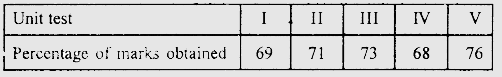Find the probability that the student gets more than $70 \%$ marks."
">

# The percentage of marks obtained by a student in monthly unit tests are given below:Find the probability that the student gets more than $70 \%$ marks."

Given:

The percentage of marks obtained by a student in monthly unit tests.

To do:

We have to find the probability that the student gets more than $70 \%$ marks.

Solution:

Total number of unit tests $=5$

Number of times the student gets more than $70 \%$ marks $=3$

We know that,

Probability of an event=$\frac{Number \ of \ favourable \ outcomes}{Total \ number \ of \ outcomes}$

Therefore,

Probability that the student gets more than $70 \%$ marks $=\frac{3}{5}$

$=0.6$

This implies,

The probability that the student gets more than $70 \%$ marks is $0.6$.

Updated on: 10-Oct-2022

45 Views